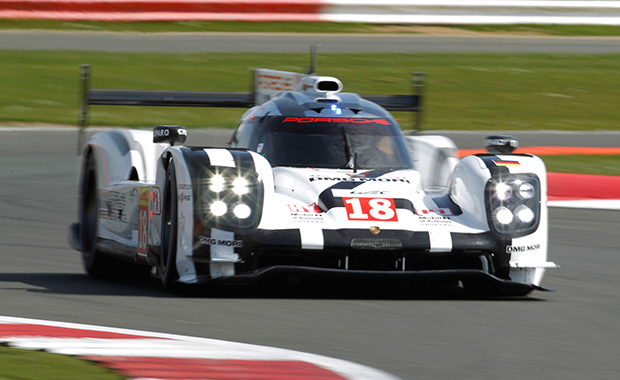# Grip# What Is Grip?

Grip, put basically, is the force generated between the tyre and the surface. The amount of grip generated and maintained is influenced heavily by the mass of the vehicle; the acceleration of the vehicle, the compound of the tyre, the compound of the road surface and by the suspension geometry of the suspension system.

The simple equation to calculate the frictive force is:

F = UN

Where:

F = Force (N)
U = Friction Co-efficient
N = Normal Force

Friction is heavily affected by lateral acceleration. The relationship between the two under simple circumstances can be seen within the friction circle diagram shown below.The friction is developed from the tyre distorting and displacing due to the forces exerted through it from the vehicle. This distortion is known as the slip angle of the tyre.

The characteristics of the slip angle are dictated by the compound of the tyre and can be analysed with the use of a slip angle graph, often provided by the tyre manufacturer showing the relationship between the slip angle and the maximum resultant force possible to be handled by the tyre.